SPSS TUTORIALS BASICS ANOVA REGRESSION FACTOR CORRELATION

# SPSS – Kendall’s Concordance Coefficient W

Kendall’s Concordance Coefficient W is a number between 0 and 1 that indicates interrater agreement. So let's say we had 5 people rank 6 different beers as shown below. We obviously want to know which beer is best, right? But could we also quantify how much these raters agree with each other? Kendall’s W does just that.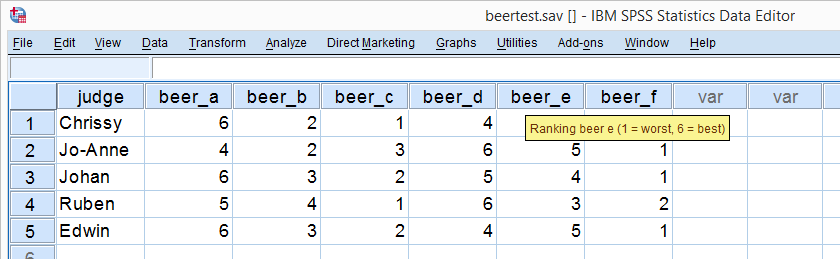## Kendall’s W - Example

So let's take a really good look at our beer test results. The data -shown above- are in beertest.sav. For answering which beer was rated best, a Friedman test would be appropriate because our rankings are ordinal variables. A second question, however, is to what extent do all 5 judges agree on their beer rankings? If our judges don't agree at all which beers were best, then we can't possibly take their conclusions very seriously. Now, we could say that “our judges agreed to a large extent” but we'd like to be more precise and express the level of agreement in a single number. This number is known as Kendall’s Coefficient of Concordance W.2,3

## Kendall’s W - Basic Idea

Let's consider the 2 hypothetical situations depicted below: perfect agreement and perfect disagreement among our raters. I invite you to stare at it and think for a minute.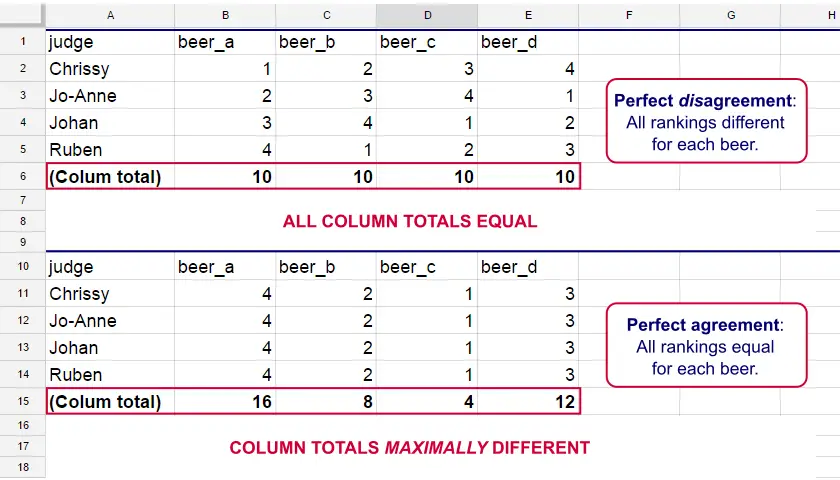As we see, the extent to which raters agree is indicated by the extent to which the column totals differ. We can express the extent to which numbers differ as a number: the variance or standard deviation.
Kendall’s W is defined as

$$W = \frac{Variance\,over\,column\,totals}{Maximum\,possible\,variance\,over\,column\,totals}$$

As a result, Kendall’s W is always between 0 and 1. For instance, our perfect disagreement example has W = 0; because all column totals are equal, their variance is zero.
Our perfect agreement example has W = 1 because the variance among column totals is equal to the maximal possible variance. No matter how you rearrange the rankings, you can't possibly increase this variance any further. Don't believe me? Give it a go then.
So what about our actual beer data? We'll quickly find out with SPSS.

## Kendall’s W in SPSS

We'll get Kendall’s W from SPSS’ Nonparametric Tests menu. The screenshots below walk you through.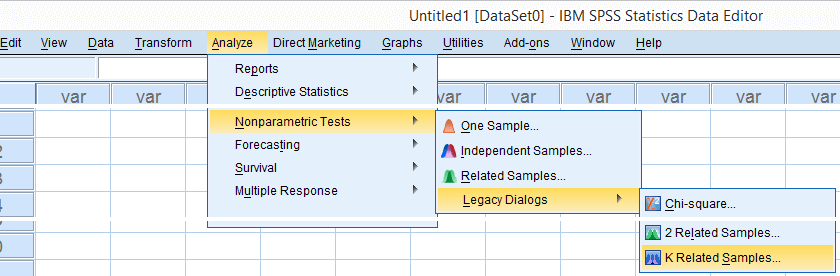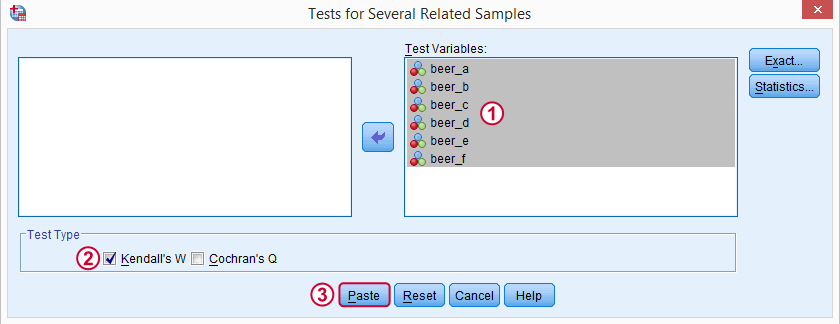Note: SPSS thinks our rankings are nominal variables. This is because they contain few distinct values. Fortunately, this won't interfere with the current analysis. Completing these steps results in the syntax below.

## Kendall’s W - Basic Syntax

*Kendall's W from nonparametric tests - legacy dialogs - k related samples.

NPAR TESTS
/KENDALL=beer_a beer_b beer_c beer_d beer_e beer_f
/MISSING LISTWISE.

## Kendall’s W - Output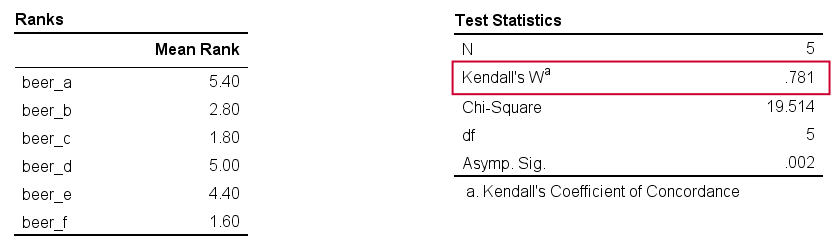And there we have it: Kendall’s W = 0.78. Our beer judges agree with each other to a reasonable but not super high extent. Note that we also get a table with the (column) mean ranks that tells us which beer was rated most favorably.

## Average Spearman Correlation over Judges

Another measure of concordance is the average over all possible Spearman correlations among all judges.1 It can be calculated from Kendall’s W with the following formula

$$\overline{R}_s = {kW - 1 \over k - 1}$$

where $$\overline{R}_s$$ denotes the average Spearman correlation and $$k$$ the number of judges. For our example, this comes down to

$$\overline{R}_s = {5(0.781) - 1 \over 5 - 1} = 0.726$$

We'll verify this by running and averaging all possible Spearman correlations in SPSS. We'll leave that for a next tutorial, however, as doing so properly requires some highly unusual -but interesting- syntax.

## References

1. Howell, D.C. (2002). Statistical Methods for Psychology (5th ed.). Pacific Grove CA: Duxbury.
2. Slotboom, A. (1987). Statistiek in woorden [Statistics in words]. Groningen: Wolters-Noordhoff.
3. Van den Brink, W.P. & Koele, P. (2002). Statistiek, deel 3 [Statistics, part 3]. Amsterdam: Boom.

# Tell us what you think!

*Required field. Your comment will show up after approval from a moderator.

# THIS TUTORIAL HAS 15 COMMENTS:

•### By Ruben Geert van den Berg on April 23rd, 2018

Hi Eduardo!

Thanks for the compliment! What do "1 to 5" mean? Like 1 = lowest sufficiency/relevance... and 5 = highest sufficiency/relevance... ?

In this case, you could just run Kendall's W for each aspect separately, right? Or am I mistunderstanding something?

•### By Eduardo on April 23rd, 2018

Ruben

Yes, the rating refers to 1 as the lowest and 5 the highest relevance. Just to know that I understood you. Do you suggest that I calculate (run) Kendall’s W for each option? That is, I will have 4 coefficient of agreement?

•### By Ruben Geert van den Berg on April 23rd, 2018

Hi Eduardo!

Yes, reporting 4 Kendall coefficients makes perfect sense to me. This is because raters may agree strongly on rather objective measures but not on less objective measures.

For instance, if some people rate colors on darkness or brightness (objective), they'll agree to a high extent (high Kendall's W).

But if they rate the same colors on attractiveness (subjective), they may not agree at all (low Kendall's W).

It would be interesting to see if agreement is higher/lower for some aspects than others.

Hope that helps!

•### By Peter DK on December 12th, 2019

Hello, does it mean by having the value of W is enough to conclude and describe how degree of relationship or agreement is strong or week?.
-How to relate the P-value calculated and normal given Pvalue
-How about the case of using the Chi squares?
•